# Data Mining - Decision Tree (DT) Algorithm

Desicion Tree (DT) are supervised Classification algorithms.

They are:

• easy to interpret (due to the tree structure)
• a boolean function (If each decision is binary ie false or true)

Decision trees extract predictive information in the form of human-understandable tree-rules. Decision Tree is a algorithm useful for many classification problems that that can help explain the model’s logic using human-readable “If…. Then…” rules.

• reliable and robust algorithm.
• simple to implement.

They can:

• work on categorical attributes,
• handle many attributes, so big p smaller n cases.

Each decision in the tree can be seen as an feature.

## Algorithm

The creation of a tree is a quest for:

• purity (only pure node: only yes or no)
• the smallest tree

At each level, choose the attribute that produces the “purest” nodes (ie choosing the attribute with the highest information gain)

Algorithm:

## Overfitting

Decision Trees are prone to overfitting:

• whereas ensemble of tree are not. See random forest
• Pruning can help: remove or aggregate sub-trees that provide little discriminatory power

Decision Trees can overfit badly because of the highly complex decision boundaries it can produce; the effect is ameliorated, but rarely completely eliminated with Pruning.

## Library

• package=FFTrees - Create, visualize, and test fast-and-frugal decision trees (FFTs). FFTs are very simple decision trees for binary classification problems. FFTs can be preferable to more complex algorithms because they are easy to communicate, require very little information, and are robust against overfitting.

## Example

### Titanic (Survive Yes or No)

``````if Ticket Class = "1" then
if Sex = "female" then Survive = "yes"
if Sex = "male" and age < 5 then Survive = "yes"
if Ticket Class = "1" then
if Sex = "female" then Survive = "yes"
if Sex = "male" then Survive = "no"
if Ticket Class = "3"
if Sex = "male" then Survive = "no"
if Sex = "female" then
if Age < 4  then Survive = "yes"
if Age >= 4 then Survive = "no"```
```

Every path from the root is a rule

## Type

### Univariate

Single tests at the nodes

### multivariate

Compound tests at the nodes

## Documentation / Reference

Recommended PagesBoolean - Function

A Boolean function can be represented as a rooted directed, acyclic graph, which consists of several decision nodes and terminal nodes. Viz: Structure: Binary_decision_diagram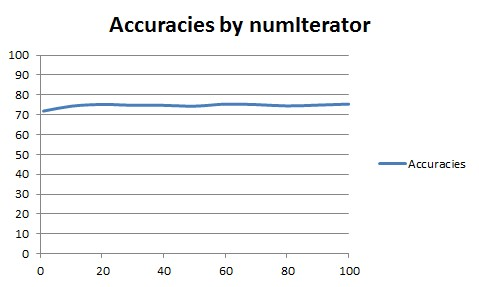Data Mining - (Boosting|Gradient Boosting|Boosting trees)

Boosting forces new classifiers to focus on the errors produced by earlier ones. boosting works by aggressively reducing the training error Gradient Boosting is an algorithm based on an ensemble of decision...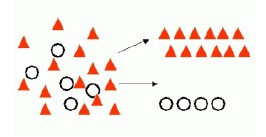Data Mining - (Classifier|Classification Function)

A classifier is a Supervised function (machine learning tool) where the learned (target) attribute is categorical (“nominal”) in order to classify. It is used after the learning process to classify...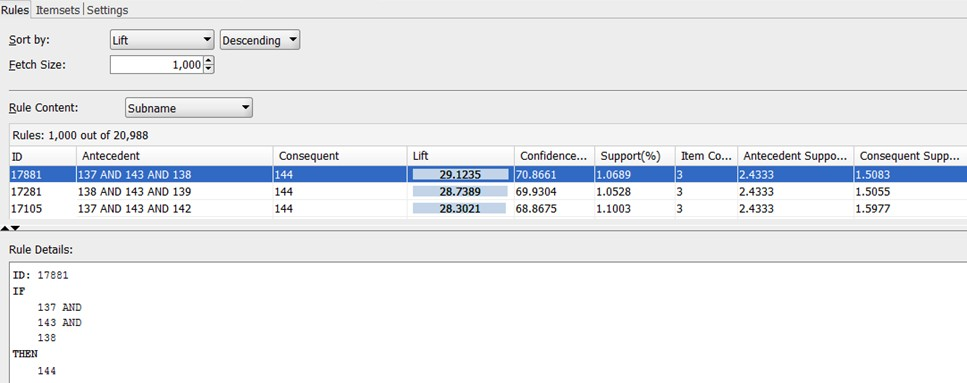Data Mining - (Decision) Rule

Some forms of predictive data mining generate rules that are conditions that imply a given outcome. Rules are if-then-else expressions; they explain the decisions that lead to the prediction. They...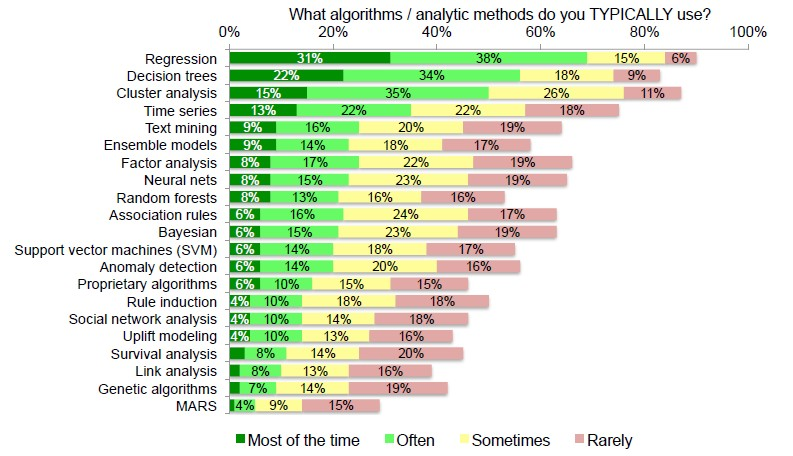Data Mining - Algorithms

An is a mathematical procedure for solving a specific kind of problem. For some data mining functions, you can choose among several algorithms. Algorithm Function Type Description Decision...Data Mining - Decision boundary Visualization

Classifiers create boundaries in instance space. Different classifiers have different biases. You can explore them by visualizing the classification boundaries. Logistic Regression method produces...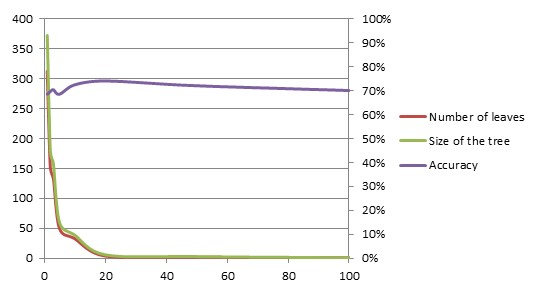Data Mining - Pruning (a decision tree, decision rules)

Pruning is a general technique to guard against overfitting and it can be applied to structures other than trees like decision rules. A decision tree is pruned to get (perhaps) a tree that generalize...Data Mining - Random forest

Random forest (or random forests) is a trademark term for an ensemble classifier that consists of many decision trees and outputs the class that is the mode of the classes output by individual trees. ...Machine Learning - (C4.5|J48) algorithm

C4.5 algorithm is a classification algorithm producing decision tree based on information theory C4.5 is from Ross Quinlan (known in Weka as J48 J for Java). He fixes ID3 to the C4.5 algorithm in 1993....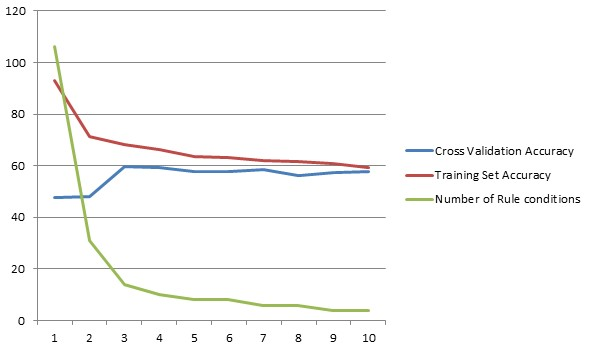Machine Learning - (One|Simple) Rule - (One Level Decision Tree)

One Rule is an simple method based on a 1‐level decision tree described in 1993 by Rob Holte, Alberta, Canada. really simple so small/noisy/complex that nothing can be learned from them ...